### ROUNDUP Function Examples – Excel, VBA, & Google Sheets

This tutorial demonstrates how to use the Excel ROUNDUP Function in Excel to round a number up.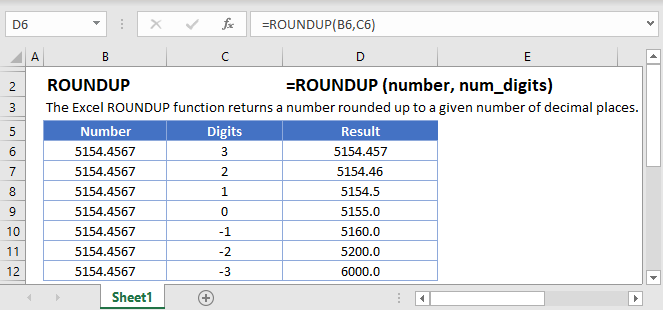## ROUNDUP Function Overview

The ROUNDUP Function Rounds a number up (away from zero) to a specified number of digits.

To use the ROUNDUP Excel Worksheet Function, select a cell and type:(Notice how the formula inputs appear)

### ROUNDUP Function Syntax and Inputs:

`=ROUNDUP(number,num_digits)`

number – A number.

num_digits – The number of decimal places to round. Negative rounds to the left of the decimal.

AutoMacro - VBA Code Generator

## How to use the ROUNDUP Function

### Round up to Nearest Whole Number

To round up to the nearest whole number set the num_digits argument to 0.

`=ROUNDUP(A2,0)`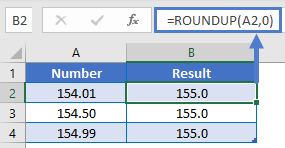### Round up to Two Decimal Places

To round up to two decimal places, set the num_digits argument to 2. Here we will round up a price to the nearest cent:

`=ROUNDUP(A2,2)`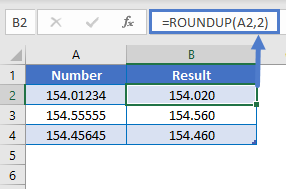### Round up to Nearest 10, 100, or 1000

To round up to the other side of the decimal (to 10, 100, or 1000s), use negative numbers for num_digits:

```=ROUNDUP(A2,-1)
=ROUNDUP(A2,-2)
=ROUNDUP(A2,-3)```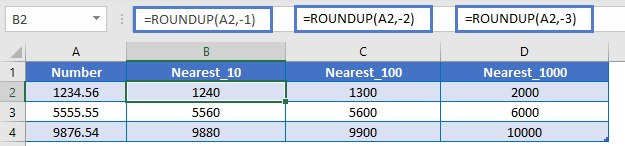### Round up Percentages

When rounding up percentages remember that percentages are decimal values. So to round up to the nearest whole percentage use the ROUNDUP Function with num_digits = 2.

`=ROUNDUP(A2,2)`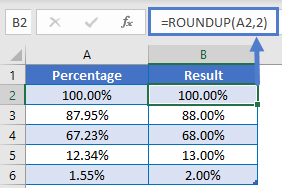## Other ROUND Functions / Formulas

Excel / Google Sheets contains many other round functions. Here are quick examples of each: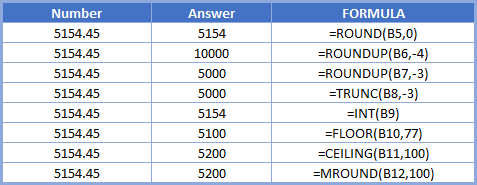Below, we will point out a few functions in particular.

### ROUND and ROUNDDOWN

The ROUND and ROUNDDOWN Functions work exactly the same way as the ROUNDUP Function, except the ROUND Function uses normal rounding rules and ROUNDDOWN always rounds down.

```=ROUND(A2,-2)
=ROUNDDOWN(A2,-2)```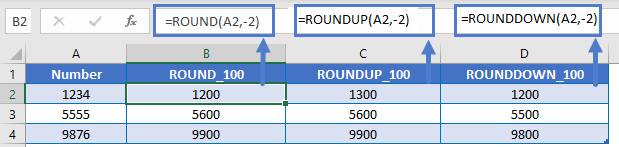### ROUND up to a Multiple

The CEILING Function is similar to the ROUNDUP Function. It will round a number up, but the CEILING Function allows you to round up to a specific multiple (instead of rounding to a specific number of digits).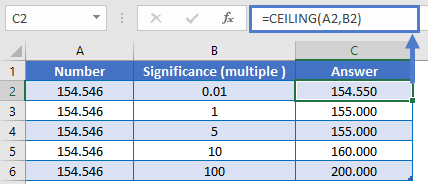The ROUNDUP Function works exactly the same in Google Sheets as in Excel: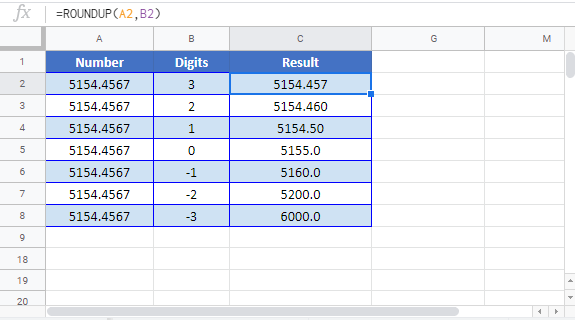## ROUNDUP Examples in VBA

You can also use the ROUNDUP function in VBA. Type:
`application.worksheetfunction.roundup(number,num_digits)`
For the function arguments (number, etc.), you can either enter them directly into the function, or define variables to use instead.